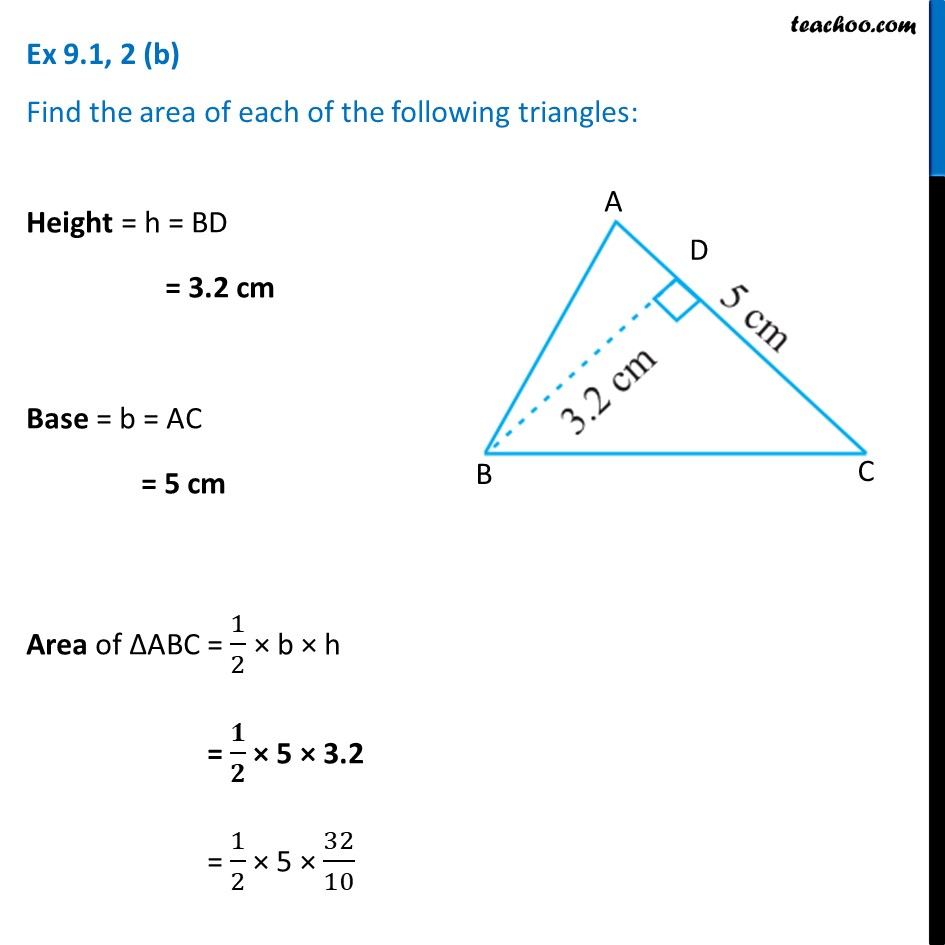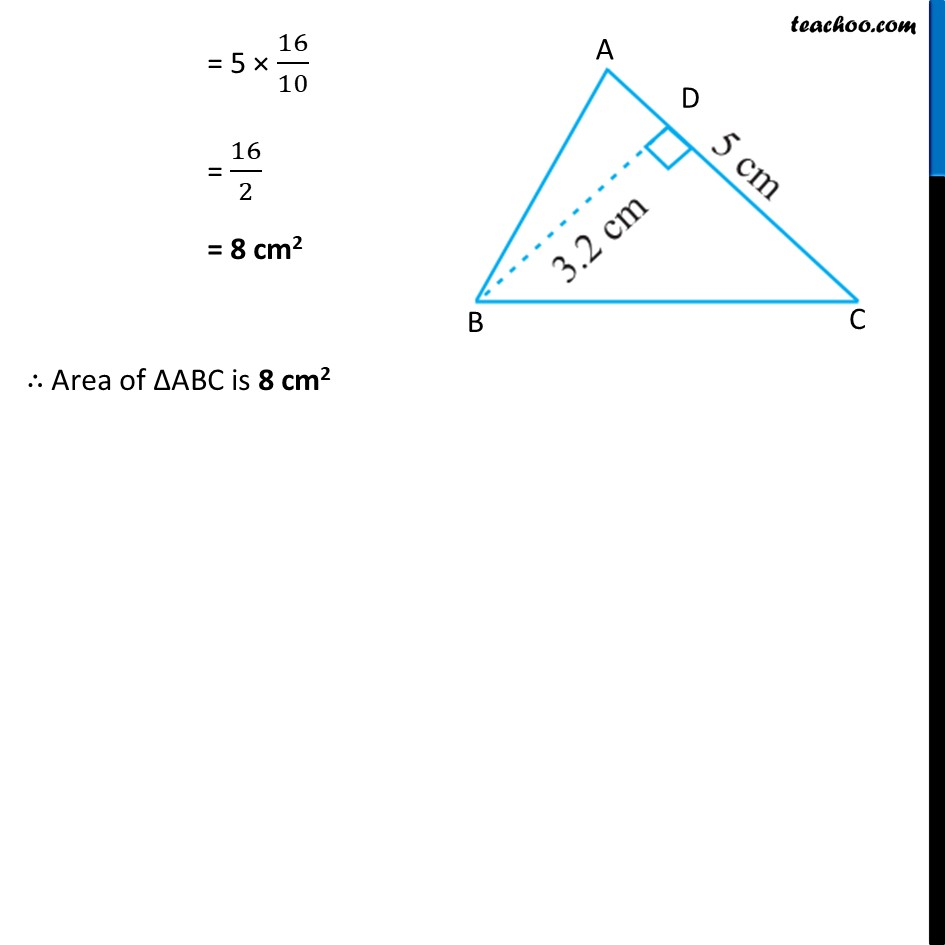Ex 9.1

Chapter 9 Class 7 Perimeter and Area
Serial order wiseLearn in your speed, with individual attention - Teachoo Maths 1-on-1 Class

### Transcript

Ex 9.1, 2 (b) Find the area of each of the following triangles: Height = h = BD = 3.2 cm Base = b = AC = 5 cm Area of ∆ABC = 1/2 × b × h = 𝟏/𝟐 × 5 × 3.2 = 1/2 × 5 × 32/10 = 5 × 16/10 = 16/2 = 8 cm2 ∴ Area of ∆ABC is 8 cm2